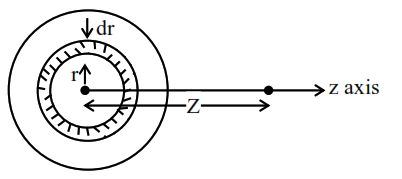# A uniformly charged

Question:

A uniformly charged disc of radius $R$ having surface charge density $\sigma$ is placed in the xy plane with its center at the origin. Find the electric field intensity along the $\mathrm{Z}$-axis at a distance $\mathrm{Z}$ from origin :-

1. $\mathrm{E}=\frac{\sigma}{2 \varepsilon_{0}}\left(1-\frac{\mathrm{Z}}{\left(\mathrm{Z}^{2}+\mathrm{R}^{2}\right)^{1 / 2}}\right)$

2. $\mathrm{E}=\frac{\sigma}{2 \varepsilon_{0}}\left(1+\frac{\mathrm{Z}}{\left(\mathrm{Z}^{2}+\mathrm{R}^{2}\right)^{1 / 2}}\right)$

3. $E=\frac{2 \varepsilon_{0}}{\sigma}\left(\frac{1}{\left(Z^{2}+R^{2}\right)^{1 / 2}}+Z\right)$

4. $E=\frac{\sigma}{2 \varepsilon_{0}}\left(\frac{1}{\left(Z^{2}+R^{2}\right)}+\frac{1}{Z^{2}}\right)$

Correct Option: 1

Solution:

Consider a small ring of radius $\mathrm{r}$ and thickness $\mathrm{dr}$ on $\operatorname{disc} .$area of elemental ring on disc

$\mathrm{dA}=2 \pi \mathrm{rdr}$

charge on this ring $\mathrm{dq}=\sigma \mathrm{dA}$

$\mathrm{dEz}=\frac{\mathrm{kdqz}}{\left(\mathrm{z}^{2}+\mathrm{r}^{2}\right)^{3 / 2}}$

$\mathrm{E}=\int_{0}^{\mathrm{R}} \mathrm{dE}_{\mathrm{z}}=\frac{\sigma}{2 \in_{0}}\left[1-\frac{\mathrm{z}}{\sqrt{\mathrm{R}^{2}+\mathrm{z}^{2}}}\right]$